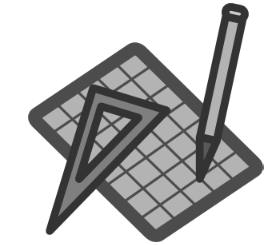## Lines and Angles (Worksheet-2)

Question: Fill in the blanks

1. Three or more points said to be __________ if there is a line which contains all these points.

2. Two lines are called ___________ lines, if they have common point.

3. Three or more lines intersecting at the same point are called as ____________ lines.

4. An angle whose measure is less than 90° is called _________ angle.

5. An angle whose measure is 90° is called _________ angle.

6. An angle whose measure is more than 90° but less than 180°, is called _______ angle.

7. An angle whose measure is more than 180° is called ______ angle.

8. If the sum of two adjacent angles is 180° then the non-common arms of the angles form a ________ _______.

9. Two angles are said to be ___________ _______, if the sum of their measure is 90°.

10. Two angles are said to ____________ _______, if the sum of their measure is 180°.

11. Two angles having a common vertex and a common arm are called __________ __________, if their common arms are on either side of the common arm.

12. If two lines intersect each other, then the _________ _________ ________ are equal.

13. If a transversal intersects two parallel lines, then each pair of corresponding angles is _________ (equal/not equal).

14. If a transversal intersects two parallel lines, then each pair of interior angles on the same side of transversal is ___________.

15. A plane figure bounded by three line segments is called a ___________.

16. The sum of three angles of a triangle is ______.

17. A triangle in which all sides are of different lengths, is called __________ triangle.

18. A triangle whose two sides are equal, is called _____________ triangle.

19. A triangle whose all sides are equal is said to be ________ triangle.

20. If a side of a triangle is produced, the exterior angle so formed is qual to the sum of _____ _______ _______ angles.

1. Collinear
2. Intersecting lines
3. concurrent
4. acute
5. right
6. obtuse
7. reflex
8. straight line.
9. complementary angles
10. suplementary angles
12. vertically opposite angles
13. equal.
14. supplementary
15. triangle
16. 180°
17. scalene
18. isoceles
19. equilateral
20. two interior opposite1.2.